The Reynolds number of a flow is the ratio of

(a) Gravity to viscous force

(b) Gravity force to pressure force

(c) Inertia forces to viscous force

(d) Viscous forces to pressure forces

Concept Questions :-

Types of flow

Difficulty Level:

Water is flowing through a tube of non-uniform cross-section ratio of the radius at entry and exit end of the pipe is 3 : 2. Then the ratio of velocities at entry and exit of liquid is -

(a) 4 : 9                          (b) 9 : 4
(c) 8 : 27                         (d) 1 : 1

Concept Questions :-

Equation of continuity
High Yielding Test Series + Question Bank - NEET 2020

Difficulty Level:

A liquid flows in a tube from left to right as shown in figure. ${\mathrm{A}}_{1}$ and ${\mathrm{A}}_{2}$ are the cross-sections of the portions of the tube as shown. Then the ratio of speeds ${\mathrm{v}}_{1}/{\mathrm{v}}_{2}$ will be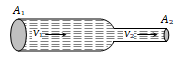(a) ${\mathrm{A}}_{1}/{\mathrm{A}}_{2}$

(b) ${\mathrm{A}}_{2}/{\mathrm{A}}_{1}$

(c) $\sqrt{{\mathrm{A}}_{2}}/\sqrt{{\mathrm{A}}_{1}}$

(d) $\sqrt{{\mathrm{A}}_{1}}/\sqrt{{\mathrm{A}}_{2}}$

Concept Questions :-

Equation of continuity

Difficulty Level:

An application of Bernoulli's equation for fluid flow is found in

(a) Dynamic lift of an aeroplane

(b) Viscosity meter

(c) Capillary rise

(d) Hydraulic press

Concept Questions :-

Bernoulli theorem

Difficulty Level:

The Working of an atomizer depends upon

(a) Bernoulli's theorem                 (b) Boyle's law
(c) Archimedes principle               (d) Newton's law of motion

Concept Questions :-

Bernoulli theorem

Difficulty Level:

The pans of a physical balance are in equilibrium. Air is blown under the right hand pan; then the right hand pan will

(a) Move up

(b) Move down

(c) Move erratically

(d) Remain at the same level

Concept Questions :-

Bernoulli theorem
High Yielding Test Series + Question Bank - NEET 2020

Difficulty Level:

According to Bernoulli's equation

$\frac{\mathrm{P}}{\mathrm{\rho g}}+\mathrm{h}+\frac{1}{2}\frac{{\mathrm{v}}^{2}}{\mathrm{g}}=\mathrm{constant}$

The terms A, B and C are generally called respectively:

4. Gravity, pressure and velocity head

Concept Questions :-

Bernoulli theorem

Difficulty Level:

A sniper fires a rifle bullet into a gasoline tank making a hole 53.0 m below the surface of gasoline. The tank was sealed at 3.10 atm. The stored gasoline has a density of 660 ${\mathrm{kgm}}^{-3}$. The velocity with which gasoline begins to shoot out of the hole is
(a) 27.8 ${\mathrm{ms}}^{-1}$                                            (b) 41.0 ${\mathrm{ms}}^{-1}$
(c)  9.6 ${\mathrm{ms}}^{-1}$                                             (d) 19.7 ${\mathrm{ms}}^{-1}$

Concept Questions :-

Bernoulli theorem
High Yielding Test Series + Question Bank - NEET 2020

Difficulty Level:

An L-shaped tube with a small orifice is held in a water stream as shown in fig. The upper end of the tube is 10.6 cm above the surface of water. What will be the height of the jet of water coming from the orifice? Velocity of water stream is 2.45 m/s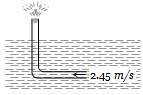(a) Zero                                         (b) 20.0 cm
(c) 10.6 cm                                    (d) 40.0 cm

Concept Questions :-

Bernoulli theorem
High Yielding Test Series + Question Bank - NEET 2020

Difficulty Level:

Fig. represents vertical sections of four wings moving horizontally in air. In which case the force is upwards

(a)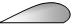(b)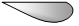(c)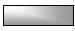(d)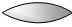Concept Questions :-

Bernoulli theorem